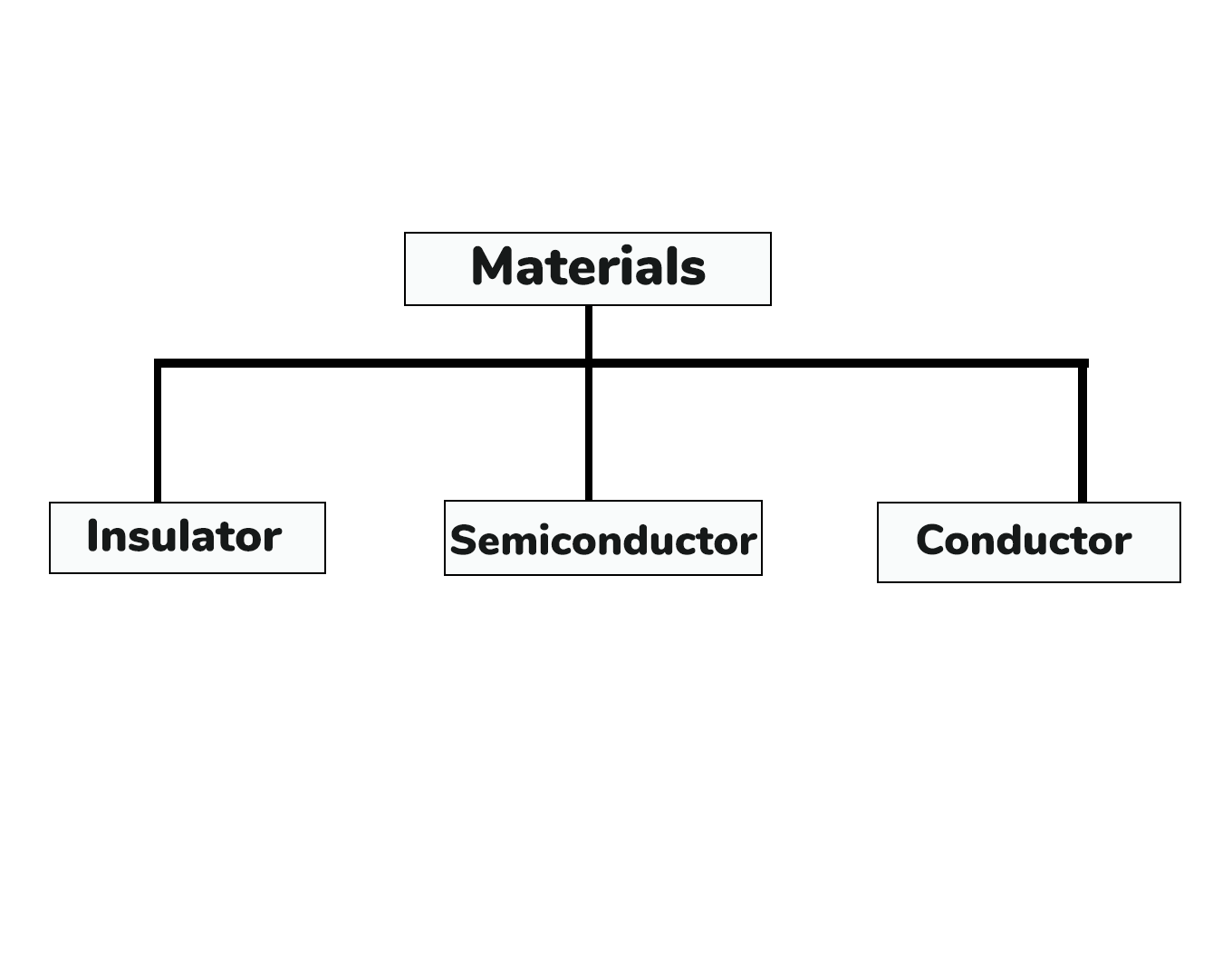### Introduction to Semiconductors

Based on the electrical conductivity all the materials in nature are classified as insulators, semiconductors, and conductors.1. Insulator:

An insulator is a material that provides little (or negligible) conductivity when a voltage is applied. For example: paper, mica, glass, quartz. Typical insulator resistance levels are around  1010 to 1012 Ω-cm. The band structure of the insulator is shown in Figure. The band structure of a material determines the range of energy levels that an electron can occupy. The valence band is the energy range of an electron in which the electron remains bent with the atom and does not contribute to an electric current. Conduction twist is the range of electron energy above the valence band where, under the influence of an external voltage source, electrons are freely accelerated resulting in a flow of charge. The energy band between the valence band and the conduction band is called the forbidden band gap. This is the energy required for an electron to move from the equilibrium band to the conduction band, that is, the energy required for a valence electron to become a free electron.

1 eV = 1.6 x 10-19 J
For insulators, there is a  forbidden band gap greater than 5Ev as shown in Figure 1.1. Because of this large gap, there are few electrons in the CB, so the insulator conducts poorly. Even an increase in temperature or an applied electric field is not sufficient to transfer electrons from VB to CB.Energy band diagram insulator, semiconductor and conductor

1. Conductor:

The conductor is a material that supports a generous charge stream when the voltage is applied to terminals. In other  words. It has a very high conductivity. Example: copper, aluminum, silver, gold. The resistivity of the conductor is about 10-4 and 10-6 Ωcm. The Valence and conduction bands are overlapping (shown in Figure) and there is no energy gap for the electrons to move from valence band to conduction band. This means that the absolute zero temperature (0k) also has free electronics in CB. As a result, when the electric field is applied at room temperature, the high current flows through conductors.

1. Semiconductor:

A semiconductor is a material that has its conductivity somewhere between the insulator and conductor. The resistivity level is in the range of 10 and 104 Ω-cm. Two of the most commonly used are Silicon (Si=14 atomic no.) and germanium (Ge=32 atomic no.). Both have 4 valence  electrons. The forbidden band gap is in the order of 1eV. For eg., the band gap energy for Si, Ge and  GaAs is 1.21, 0.785 and 1.42 eV, respectively at absolute zero temperature (0K). At 0K and at low
temperatures, the valence band electrons do not have sufficient energy to move from V to CB.Thus semiconductors act as insulators at 0K. As the temperature increases, a large number of valence electrons acquire sufficient energy to leave the VB, cross the forbidden band gap and reach CB.These are now free electrons as they can move freely under the influence of electric field. At room temperature there are sufficient electrons in the CB and hence the semiconductor is capable of conducting some current at room temperature

Inversely proportional to the conductivity of a material is its resistance to charge flow or current.Typical resistivity values for various materials’ are given as follows.

 Insulator Semiconductor Conductor 10-6 Ω-cm (Cu) 50 Ω-cm (Ge) 1012 Ω-cm (mica) 50x103 Ω-cm (Si)